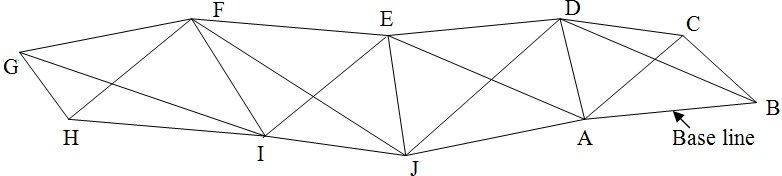Feb 26, 2016

Feb 26, 2016

Feb 26, 2016

# What is Trilateration Surveying

## Trilateration:

Because of the development of highly accurate electronic measuring devices, a triangulation system can be completely observed, computed and adjusted by measuring the lengths o the sides in the network. This procedure is known as trilateration. No horizontal angle need to be measured because the lengths of the sides are sufficient to permit both the horizontal angles and the positions of the stations to be computed.

The surveying solution technique of measuring only the side of triangle is called triplication.Trilateration surveying is a technique used in surveying and geodesy to determine the position or location of a point by measuring distances to known reference points. It is commonly used in GPS (Global Positioning System) technology and other positioning systems.

Trilateration involves measuring the distances between the point of interest and at least three known reference points with known coordinates. These reference points are typically established using more accurate surveying methods, such as GPS or total station measurements. The distances can be measured using various techniques, such as electronic distance measurement (EDM) devices or tape measures.

Once the distances to the reference points are measured, trilateration calculations are performed to determine the coordinates of the unknown point. This is done by intersecting circles or spheres centered on each reference point with radii equal to the measured distances. The point of intersection of these circles or spheres represents the location of the unknown point.

Trilateration can be performed in both two-dimensional (2D) and three-dimensional (3D) space, depending on the requirements of the survey. In 2D trilateration, the reference points and the unknown point lie on a flat plane, while in 3D trilateration, the points are located in three-dimensional space.

Trilateration surveying is widely used in various applications, including land surveying, geodesy, navigation systems, and positioning technologies like GPS. It provides an efficient and accurate method for determining the position of a point based on distance measurements to known reference points.